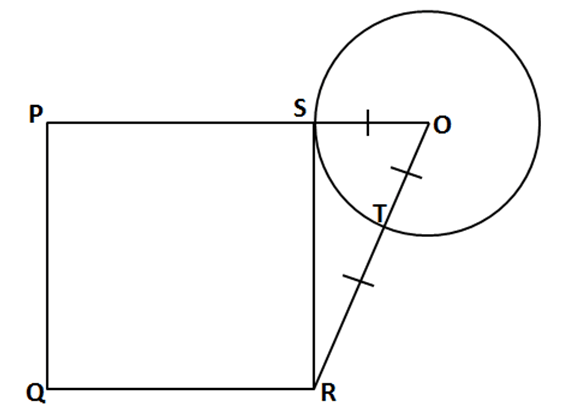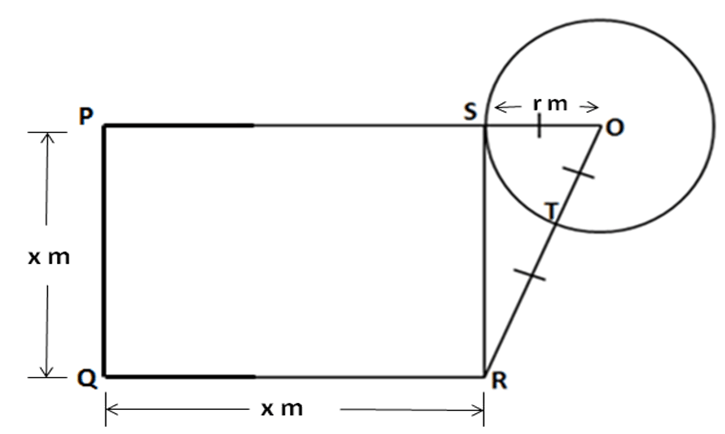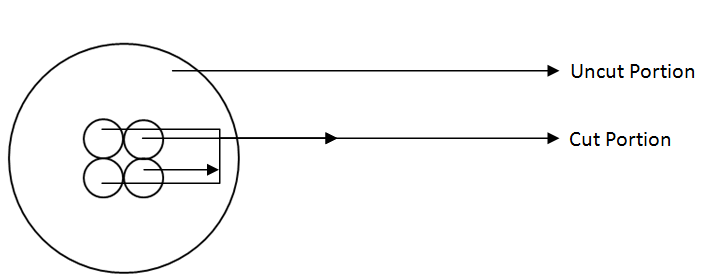Select Page

### CAT Mensuration Practice Exercise-1

CAT Mensuration problems are mainly based on the area, volume and related parameters of various shapes in geometry. Applying the formulas is the easy part, but some complex mensuration problems consist of a combination of different shapes rather than similar ones. Practice of such problems is essential to crack this type of questions when you face them in the exam.

Question 1: The sides of a triangle are 5, 12 and 13 units. A rectangle is constructed, which is equal in area to the triangle, has a width of 10 units. Then, the perimeter of the rectangle is:
(a) 30 units
(b) 36 units
(c) 13 units
(d) None of these

5,12 and 13 are Pythagoras triplets. Hence the triangle so formed will be a right angled triangle.

Greatest side is the hypotenuse which is 13, and with 12 as height and 5 as base.

Area of triangle = ½ × Base × Height = ½ × 12 × 5 = 30 sq units.

Area of rectangle = Area of Triangle = 30 sq units Area of rectangle = length × breadth = 30.

Given breadth or width = 10 units Therefore, length × 10 = 30 =>length = 3 units.

Perimeter of the rectangle = 2 × (length + breadth) = 2 × (3 + 10) = 2 × 13 = 26 units.

Hence the perimeter of the rectangle is 26 units.

Question 2: PQRS is a square. SR is a tangent (at point S) to the circle with centre O and TR= OS. Then, the ratio of area of the circle to the area of the square is:(a) π/3
(b) 11/7
(c) 3/ π
(d) 7/11

Let x m be the side of the square => PQ = QR = RS = SP = x m

Let r be the radius of the circle => OS = OT = r m

Then we have following –Now Given TR = OT = r m

OR = OT + TR

= r + r

= 2r

Area of the circle is πr2

Area of Square is x2

Now in triangle RSO,

OS2 + SR2 = OR2

r2 + x2 = (2r)2 = 4r2

x2 = 3r2

So area of Square PQRS= x2 = 3r2

Therefore the ratio of area of the circle to the area of the square is  = πr2 /3r2 =π/3

Question 3: Which one of the following cannot be the ratio of angles in a right-angled triangle?

(a) 1: 2: 3
(b) 1: 1: 2
(c) 1: 3: 6
(d) None of these

We can solve the answer by using the options provided to us. We know that the sum of angles of a triangle is 180˚ and in a right angled triangle the largest angle is 90˚. Using these two facts we shall evaluate the options given –

Option (a) 1: 2: 3 => Angles are x, 2x and 3x. Sum = 6x = 180

=> x = 30. Angles are 30, 60 and 90. Hence it is a right angle triangle.

Option (b) 1: 1: 2 => Angles are x, x and 2x. Sum = 4x = 180

=> x = 45. Angles are 45, 45 and 90.

Hence it is a right angle triangle.

Option (c) 1: 3: 6 => Angles are x, 3x and 6x.

Sum = 10x = 180 => x = 18.

Angles are 18, 54 and 108. Here none of the angles is of 900, therefore this cannot be the ratio of angles of a right angled triangle.

Question 4: A wooden box (open at the top) of thickness 0·5 cm, length 21 cm, width 11 cm and height 6 cm is painted on the inside. The expenses of painting are Rs.70. What is the rate of painting per square centimeter?

(a) Re. 0·7
(b) Re 0·5
(c) Re 0·1
(d) Re 0·2

The dimensions given to us are from outside, and the box is painted from inside, we need to find the dimensions of the inside of the box.

Thickness = 0.5 cm

The thickness needs to be subtracted for getting internal dimensions.

length = 21 cm

Inside length = length – 2 × thickness = 21 – 2 × 0.5 = 21 – 1 = 20 cm

Inside breadth = Breadth – 2 × thickness = 11 – 2 × 0.5 = 11 – 1 = 10 cm

Height = 6 cm

Inside height = Height – thickness = 6 – 0.5 = 5.5 cm

(Note: it is an open box, so thickness from height is subtracted only once)

Total Area to be painted = Area of 4 side walls + Area of the base

Area of 4 walls = 2 × height × (length + breadth) = 2 × 5.5 × (20 + 10) = 330 sq cm

Area of Base = length × breadth = 20 × 10 = 200 sq cm

Total area to be painted = 530 sq cm

Cost of painting = Rs. 70

Cost per sq cm = 70/530 = 0.13 or Re 0.1 per sq. cm (approx)

Question 5: From a circular sheet of paper with a radius 20 cm, four circles of radius 5 cm each are cut out. What is the ratio of the uncut to the cut portion?

(a) 1: 3
(b) 4: 1
(c) 3: 1
(d) 4: 3

Following is the representation of the question given above,Area of Circle is π R2

Area of sheet of paper with radius 20 cm π (20)2 = 400 πcm2

Area of cut portion = Area of 4 circles of radius 5 cm = 4π(5)2  = 100 πcm2

Area of uncut portion = Area of sheet of paper – Area of cut portion

Therefore, Area of uncut portion = 400 π-100 π = 100 πcm2

Therefore, Ratio of area of uncut portion to area of cut portion

300 π:100 π= 3:1

Extra tips for CAT Mensuration Problems:

• While solving CAT Mensuration Problems, you will encounter questions where 2 or more different shapes are involved. In this case, try sketching a rough diagram and finding the relation between the various shapes and their intersections. For this, you need analyze the question thoroughly.
• Revise the concepts like Pythagoras theorems, internal and external angles, properties of various types of triangles, etc.
• The simplest method to proceed in such complex problems is to find the common side of the given shape and then find the related parameters.
• Be careful while defining different variables as multiple shapes are involved which may contain similar parameters.
Get Posts Like This Sent to your Email
Updates for Free Live sessions and offers are sent on mail. Don't worry: we do not send too many emails..:)
Get Posts Like This Sent to your Email
Updates for Free Live sessions and offers are sent on mail. Don't worry: we do not send too many emails..:)